AI工具箱## 构建高质量机器学习数据集的初学者指南

2023年11月17日 由 alex 发表 44 0

`pip install ydata-profiling==4.6.1`

`import pandas as pdfrom ydata_profiling import ProfileReportdf = pd.read_csv('diabetes.csv')# Generate the reportprofile = ProfileReport(df,title="Diabetes Data Profile")# Save the report to .htmlprofile.to_file("diabetes_report.html")`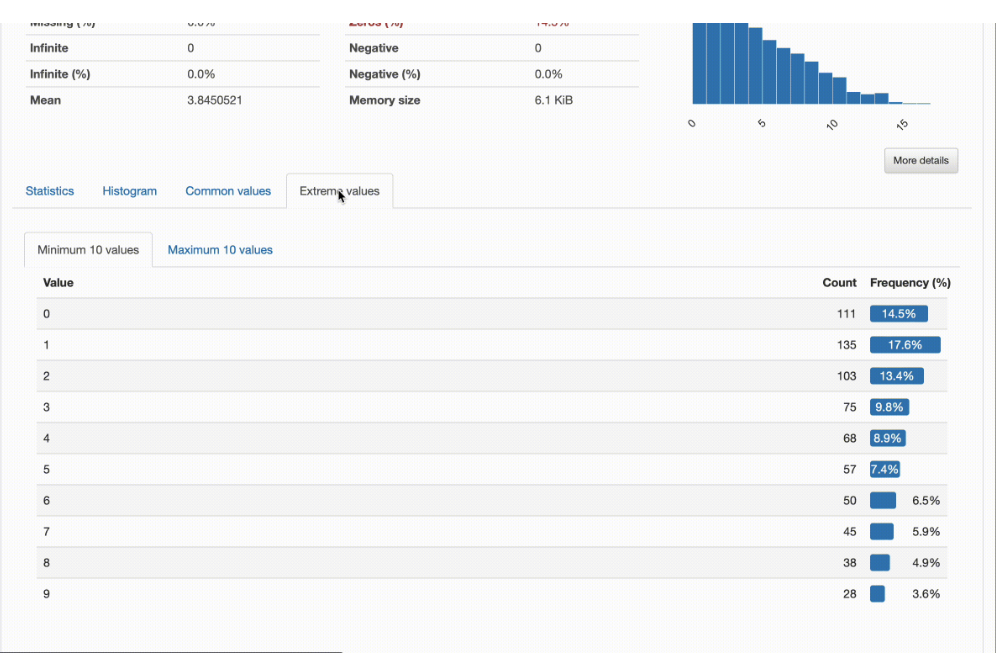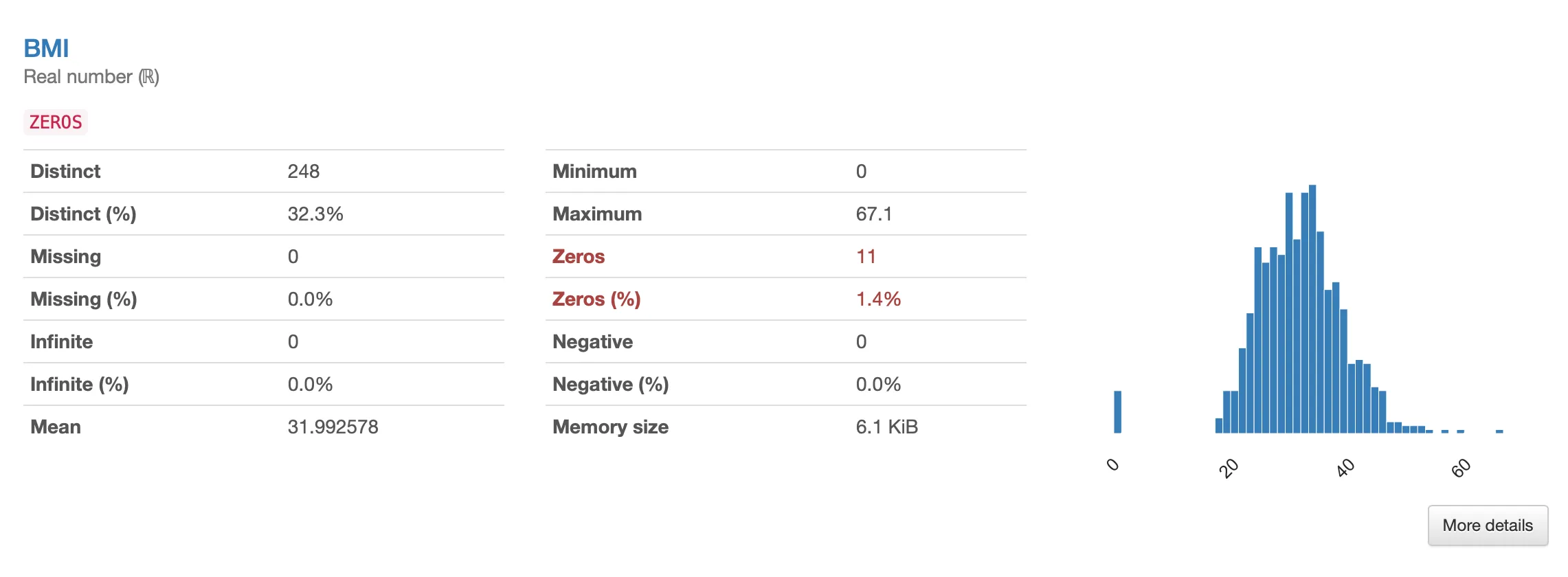`import numpy as npdf[['Glucose', 'BloodPressure', 'SkinThickness', 'Insulin', 'BMI']] = df[['Glucose', 'BloodPressure', 'SkinThickness', 'Insulin', 'BMI']].replace(0, np.nan)`

`from sklearn.model_selection import train_test_splitfrom sklearn.impute import SimpleImputer# Split data into training and testX = df.iloc[:,:-1]y = df.iloc[:,-1]X_train, X_test, y_train, y_test = train_test_split(X, y, test_size=0.2)# Perform data imputationimputer = SimpleImputer(strategy='mean')imputer.fit(X_train)X_train = imputer.transform(X_train)X_train = pd.DataFrame(X_train, columns=df.columns[:-1])X_test = imputer.transform(X_test)X_test = pd.DataFrame(X_test, columns=df.columns[:-1])# Keep integer values in selected columnsX_train[['Glucose', 'BloodPressure', 'SkinThickness', 'Insulin', 'BMI']] = X_train[['Glucose', 'BloodPressure', 'SkinThickness', 'Insulin', 'BMI']].round(0)X_test[['Glucose', 'BloodPressure', 'SkinThickness', 'Insulin', 'BMI']] = X_test[['Glucose', 'BloodPressure', 'SkinThickness', 'Insulin', 'BMI']].round(0)`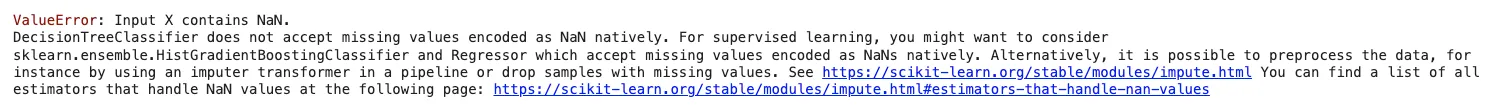`from sklearn.tree import DecisionTreeClassifierfrom sklearn.metrics import accuracy_score, classification_reportfrom sklearn.metrics import confusion_matrixclf = DecisionTreeClassifier()clf.fit(X_train, y_train)y_pred = clf.predict(X_test)report = classification_report(y_test, y_pred)print("Classification Report:\n", report)conf_matrix = confusion_matrix(y_test, y_pred)print(conf_matrix)这是混淆矩阵：array([[73, 26],       [26, 29]])`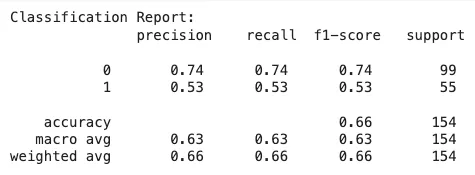`array([[73, 26],       [26, 29]])`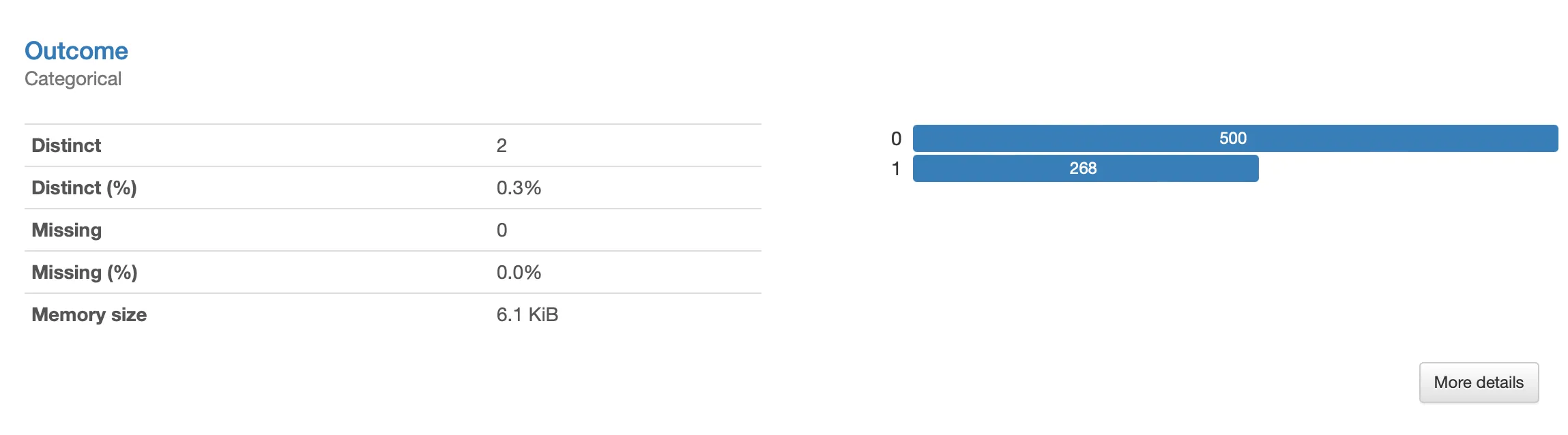`pip install ydata-synthetic==1.3.1`

`from ydata_synthetic.synthesizers.regular import RegularSynthesizerfrom ydata_synthetic.synthesizers import ModelParameters, TrainParametersX_train.reset_index(drop=True, inplace=True)y_train = pd.DataFrame(y_train, columns=[df.columns[-1]])y_train.reset_index(drop=True, inplace=True)train_df = pd.concat([X_train, y_train], axis=1)num_cols = list(df.columns[:-1])cat_cols = [df.columns[-1]] synth = RegularSynthesizer(modelname='fast')synth.fit(data=train_df, num_cols=num_cols, cat_cols=cat_cols)# Generate 1000 samplessynth_data = synth.sample(1000)# Keep newly generated values aligned with the range and format of original featuressynth_data[['Pregnancies', 'Glucose', 'BloodPressure', 'SkinThickness', 'Insulin', 'BMI', 'Age']] = synth_data[['Pregnancies', 'Glucose', 'BloodPressure', 'SkinThickness', 'Insulin', 'BMI', 'Age']].applymap(lambda x: max(round(x, 0), 0))synth_data[['DiabetesPedigreeFunction']] = synth_data[['DiabetesPedigreeFunction']].applymap(lambda x: max(x, 0))`

`min_df = synth_data[synth_data['Outcome']==1]min_df.reset_index(drop=True, inplace=True)min_sample_df = min_df.sample(188).reset_index(drop=True)synth_df = pd.concat([train_df, min_sample_df], axis=0)`

`clf = DecisionTreeClassifier()X_aug = synth_df.iloc[:, :-1]y_aug = synth_df.iloc[:,-1]clf.fit(X_aug, y_aug)y_pred = clf.predict(X_test)# Classification resultsreport = classification_report(y_test, y_pred)print("Classification Report:\n", report)conf_matrix = confusion_matrix(y_test, y_pred)print(conf_matrix)`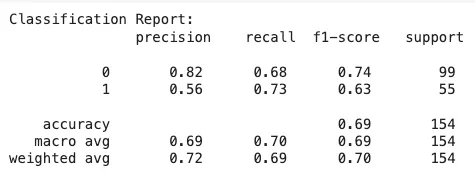`array([[67, 32],       [15, 40]])`AI热点
AI工具

Maluuba
20000~40000/月
Cisco
25000~30000/月 深圳市
PilotAILabs
30000~60000/年 深圳市